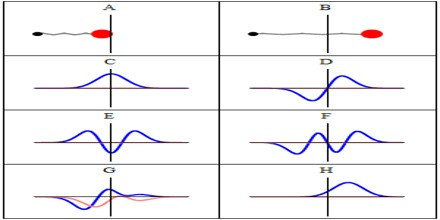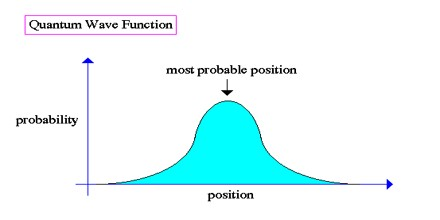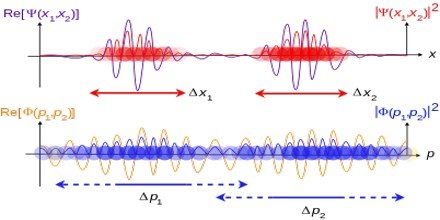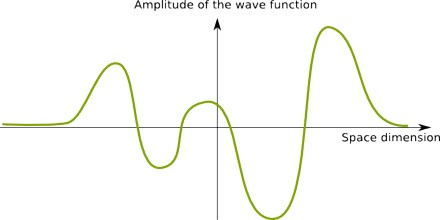Defionition

A wave function is a function that encodes the state of a quantum-mechanical system. Typically the wave function obeys a wave equation or modified wave equation that has wave-like solutions, hence the name. The wave function is a function of the degrees of freedom corresponding to some maximal set of commuting observables. Once such a representation is chosen, the wave function can be derived from the quantum state.Wave function, in quantum mechanics, variable quantity that mathematically describes the wave characteristics of a particle. The value of the wave function of a particle at a given point of space and time is related to the likelihood of the particle’s being there at the time. By analogy with waves such as those of sound, a wave function, designated by the Greek letter psi, Ψ, may be thought of as an expression for the amplitude of the particle wave (or de Broglie wave), although for such waves amplitude has no physical significance.

The quantum wavefunction is a way of representing the physical possibilities within quantum physics. The wavefunction is something called a “state vector” and it represents the physical parameters of a particle (or a set of particles) as a set of numbers. In a real sense, the particles themselves are the set of numbers described by the quantum wavefunction.

The wavefunction equation was developed by Erwin Schrodinger, and so it is called the Schrodinger equation.

The wave function is a solution to Schrödinger’s equation, given the boundary conditions that describe the physical system in which the particle is found. The square of the absolute value of the function evaluated at a given point in space is proportional to the probability of finding the particle in the immediate vicinity of that position.

Quantum Wavefunction

The quantum wavefunction is not strictly speaking a result of quantum physics, but rather one of the fundamental concepts upon which the mathematical formalism of quantum physics is built. The existence of the quantum wavefunction is assumed and then its reality is demonstrated by the fact that making this assumption leads to predictions which conform well to physical reality through the results of quantum experiments and measurements of quantum phenomena.The wavefunction is a complex function, which is a function containing quantities that are complex numbers and is constructed in such a way that performing an operation to take the modulus of the complex function for a given state results in a real value that represents the probability density of a result that corresponds to that state. The process of transitioning from a general quantum wavefunction into a definite value for a specific state is typically called the “collapse of the wavefunction.”

Why the Wave function is not a Physical Field?

If the wave function is a physical field, then its mass and charge density will simultaneously distribute in space. This has two disaster consequences at least. One is that charge will not be quantized; the total charge inside a very small region can be much smaller than an elementary charge for a single quantum system. This obviously contradicts the common expectation that charge should be quantized. But maybe our expectation needs to be revised. So this consequence is not fatal for the field explanation of the wave function. The other is that the wave function will not satisfy the superposition principle. For example, for the wave function of a single electron, different spatial parts of the wave function will have gravitational and electrostatic interactions, as these parts have mass and charge simultaneously.As another example, consider the electron in the hydrogen atom. Since the potential of its electrostatic self-interaction is of the same order as the Coulomb potential produced by the nucleus, the energy levels of hydrogen atoms will be significantly different from those predicted by quantum mechanics and confirmed by experimental observations. Therefore, the electrostatic self-interaction cannot exist for the wave function of a charged quantum system.

Since the field explanation of the wave function entails the existence of such electrostatic self-interactions, it cannot be right, i.e. the wave function cannot be a description of a physical field.

The Wave function as a Description of Quantum Motion of Particles

The failure of classical ergodic models does not exclude all possible ergodic motion of particles. If the motion of a particle is not continuous but discontinuous and random, and the probability density of the particle being in certain positions is proportional to the square of the absolute value of its wave function at every instant, then the particle can readily move throughout all possible regions where the wave function is nonzero during an arbitrarily short time interval near a given instant. This will solve the problems plagued by the classical ergodic models. The discontinuous ergodic motion requires no existence of a finite time scale. A particle undergoing discontinuous motion can also move from one region to another spatially separated region, no matter whether there is an infinite potential wall between them.Besides, discontinuous motion can also solve the problems of infinite velocity and accelerating radiation. The reason is that no classical velocity and acceleration can be defined for discontinuous motion, and energy and momentum will require new definition and new understanding as in quantum mechanics. Thus it seems that the discontinuous ergodic motion of particles can in principle generate the effective mass and charge density measurable by protective measurement, and thus the wave function is probably a description of such random discontinuous motion of particles.